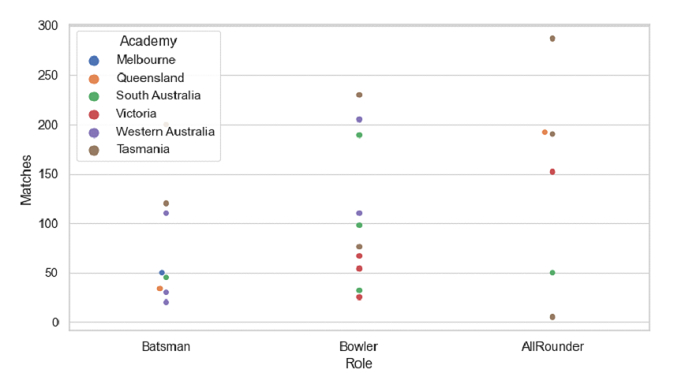# Python Pandas - Group the swarms by two categorical variables with Seaborn

Swarm Plot in Seaborn is used to draw a categorical scatterplot with non-overlapping points. The seaborn.swarmplot() is used for this. To group the swarms by two categorical variables, set those variables in the swarmplot() using the x, y or hue parameters.

Let’s say the following is our dataset in the form of a CSV file: Cricketers2.csv

At first, import the required libraries −

import seaborn as sb
import pandas as pd
import matplotlib.pyplot as plt

Load data from a CSV file into a Pandas DataFrame −

dataFrame = pd.read_csv("C:\Users\amit_\Desktop\Cricketers2.csv")


Group the swarms by two categorical variables −

sb.swarmplot(x = "Role", y = "Matches", hue = "Academy", data = dataFrame)

## Example

Following is the code

import seaborn as sb
import pandas as pd
import matplotlib.pyplot as plt

# Load data from a CSV file into a Pandas DataFrame:

# set the theme
sb.set_theme(style="whitegrid")

sb.swarmplot(x = "Role", y = "Matches", hue = "Academy", data = dataFrame)

# display
plt.show()

## Output

This will produce the following output